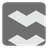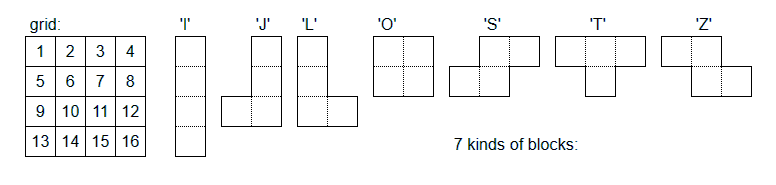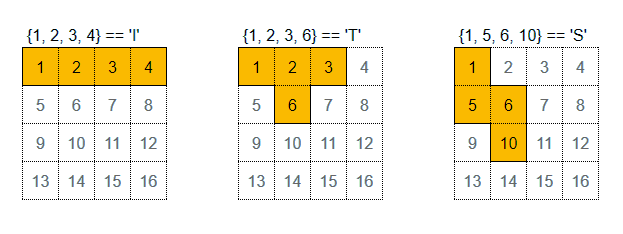Identify Block
Simple+
English RU

This mission uses a 4x4 grid. Each square is numbered from 1 to 16 in row-major order.
You are given 4 numbers (a list of integers). These numbers represent the positions of each square on the grid and a whole block if all the squares are adjacent.

You have to identify the kind of block. (Refer to the following image or comment of initial code for the kind of block).
The answer is an upper-case alphabet letter ('I', 'J', 'L', 'O', 'S', 'T' or 'Z'). If it’s not a block, then return null.

The block is placed anywhere on the grid and can be rotated (0, 90, 180 or 270 degrees).Input: 4 numbers (a list of integers)

Output: the kind of block (an alphabet letter or null )

Example:

```identifyBlock([1, 2, 3, 4]) === 'I'
identifyBlock([1, 2, 3, 6]) === 'T'
identifyBlock([1, 5, 6, 10]) === 'S'
```How it is used:
For creating a tetromino puzzle.

Precondition:

• len(input) == 4
• all(1 <= num <= 16 for num in input)
• output in ('I', 'J', 'L', 'O', 'S', 'T', 'Z', null )